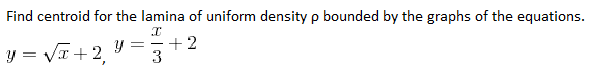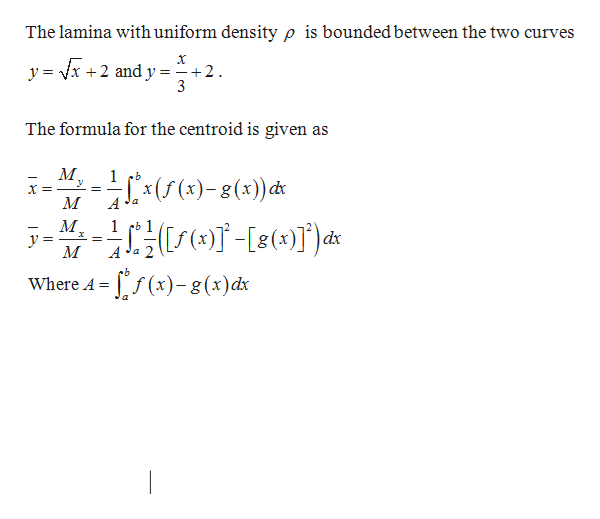# Find centroid for the lamina of uniform density p bounded by the graphs of the equations.+23y= VI+2

Question
6 views

choose the correct option

a) (18/5,7/2)   b) (9/2,7/2) c) (4,3) d) (3,4)help_outlineImage TranscriptioncloseFind centroid for the lamina of uniform density p bounded by the graphs of the equations. +2 3 y= VI+2 fullscreen
check_circle

Step 1

Given:help_outlineImage TranscriptioncloseThe lamina with uniform density p is boundedbetween the two curves y = vx +2 and y = 2 The formula for the centroid is given as 1 М 1 dx У A -/)-g()d Where A = fullscreen
Step 2

First find the limit points.

Step 3

Find the value of ...

### Want to see the full answer?

See Solution

#### Want to see this answer and more?

Solutions are written by subject experts who are available 24/7. Questions are typically answered within 1 hour.*

See Solution
*Response times may vary by subject and question.
Tagged in

### Math